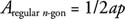## Regular Polygons

Thus far, we have dealt with polygons of three and four sides. But there is really no limit to the number of sides a polygon may have. The only practical limit is that unless you draw them on a very large sheet of paper, after about 20 sides or so, the polygon begins to look very much like a circle.

## Parts of a regular polygon

In a regular polygon, there is one point in its interior that is equidistant from its vertices. This point is called the center of the regular polygon. In Figure 1O is the center of the regular polygon.Figure 1 Center, radius, and apothem of a regular polygon.

The radius of a regular polygon is a segment that goes from the center to any vertex of the regular polygon.

The apothem of a regular polygon is any segment that goes from the center and is perpendicular to one of the polygon's sides. In Figure OC is a radius and OX is an apothem.

## Finding the Perimeter

Because a regular polygon is equilateral, to find its perimeter you need to know only the length of one of its sides and multiply that by the number of sides. Using n‐gon to represent a polygon with n sides, and s as the length of each side, produces the following formula.If p represents the perimeter of the regular polygon and a represents the length of its apothem, the following formula can eventually be shown to represent its area.Example 1: Find the perimeter and area of the regular pentagon in Figure 2, with apothem approximately 5.5 in.Figure 2 Finding the perimeter and area of a regular pentagon.